# Support Vector Machine (SVM) Tutorial: Learning SVMs From Examples

This post was originally published on this siteAfter the Statsbot team published the post about time series anomaly detection, many readers asked us to tell them about the Support Vector Machines approach. It’s time to catch up and introduce you to SVM without hard math and share useful libraries and resources to get you started.

If you have used machine learning to perform classification, you might have heard about Support Vector Machines (SVM). Introduced a little more than 50 years ago, they have evolved over time and have also been adapted to various other problems like regression, outlier analysis, and ranking.

SVMs are a favorite tool in the arsenal of many machine learning practitioners. At 7, we too use them to solve a variety of problems.

In this post, we will try to gain a high-level understanding of how SVMs work. I’ll focus on developing intuition rather than rigor. What that essentially means is we will skip as much of the math as possible and develop a strong intuition of the working principle.

### The Problem of Classification

Say there is a machine learning (ML) course offered at your university. The course instructors have observed that students get the most out of it if they are good at Math or Stats. Over time, they have recorded the scores of the enrolled students in these subjects. Also, for each of these students, they have a label depicting their performance in the ML course: “Good” or “Bad.”

Now they want to determine the relationship between Math and Stats scores and the performance in the ML course. Perhaps, based on what they find, they want to specify a prerequisite for enrolling in the course.

How would they go about it? Let’s start with representing the data they have. We could draw a two-dimensional plot, where one axis represents scores in Math, while the other represents scores in Stats. A student with certain scores is shown as a point on the graph.

The color of the point — green or red — represents how he did on the ML course: “Good” or “Bad” respectively.

This is what such a plot might look like: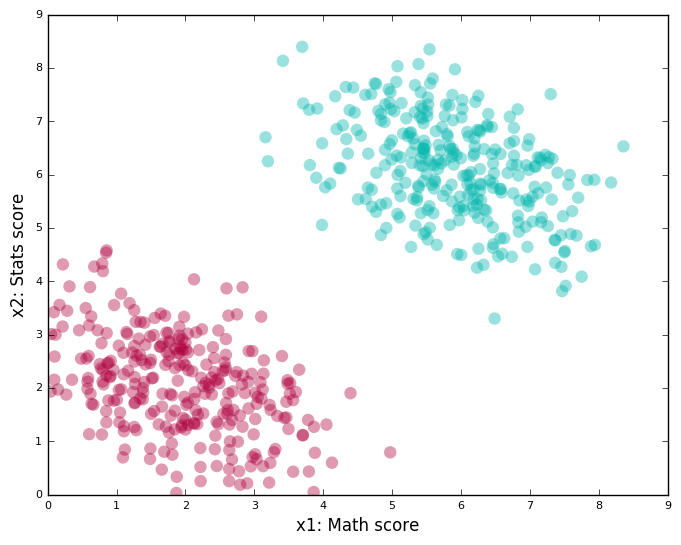When a student requests enrollment, our instructors would ask her to supply her Math and Stats scores. Based on the data they already have, they would make an informed guess about her performance in the ML course.

What we essentially want is some kind of an “algorithm,” to which you feed in the “score tuple” of the form (math_score, stats_score). It tells you whether the student is a red or green point on the plot (red/green is alternatively known as a class or label). And of course, this algorithm embodies, in some manner, the patterns present in the data we already have, also known as the training data.

In this case, finding a line that passes between the red and green clusters, and then determining which side of this line a score tuple lies on, is a good algorithm. We take a side — the green side or the red side — as being a good indicator of her most likely performance in the course.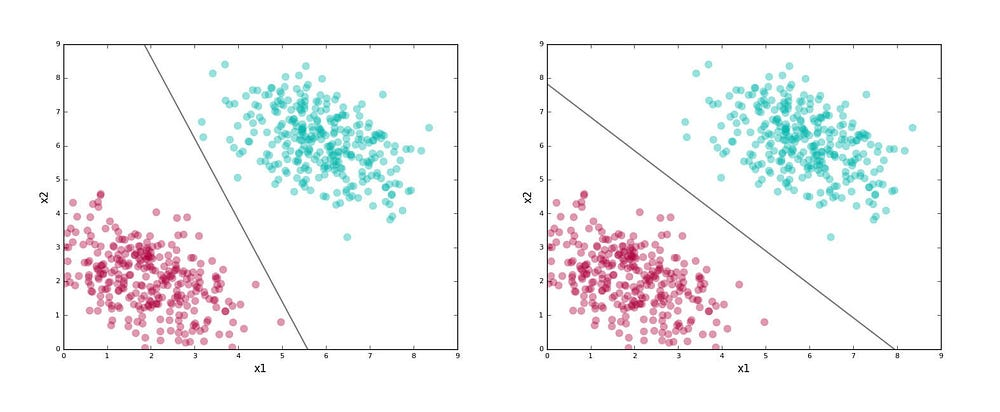The line here is our separating boundary (because it separates out the labels)or classifier (we use it classify points). The figure shows two possible classifiers for our problem.

Here’s an interesting question: both lines above separate the red and green clusters. Is there a good reason to choose one over another?

Remember that the worth of a classifier is not in how well it separates the training data. We eventually want it to classify yet-unseen data points (known as test data). Given that, we want to choose a line that captures the general pattern in the training data, so there is a good chance it does well on the test data.

The first line above seems a bit “skewed.” Near its lower half it seems to run too close to the red cluster, and in its upper half it runs too close to the green cluster. Sure, it separates the training data perfectly, but if it sees a test point that’s a little farther out from the clusters, there is a good chance it would get the label wrong.

The second line doesn’t have this problem. For example, look at the test points shown as squares and the labels assigned by the classifiers in the figure below.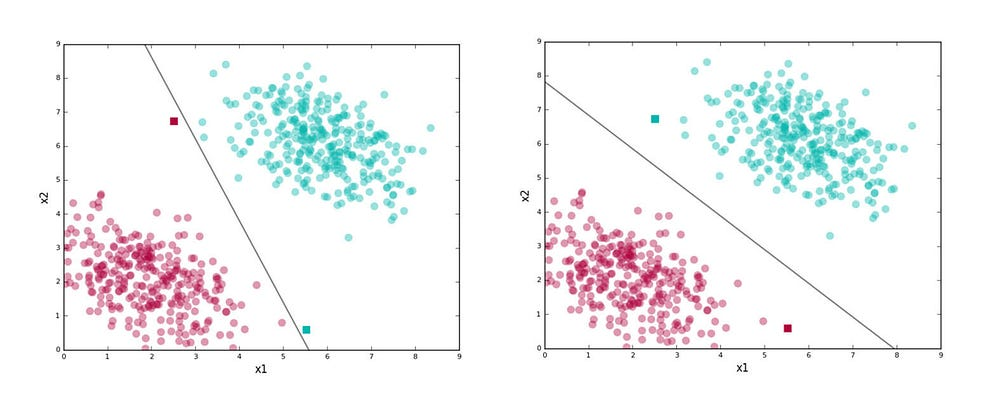The second line stays as far away as possible from both the clusters while getting the training data separation right. By being right in the middle of the two clusters, it is less “risky,” gives the data distributions for each class some wiggle room so to speak, and thus generalizes well on test data.

SVMs try to find the second kind of line. We selected the better classifier visually, but we need to define the underlying philosophy a bit more precisely to apply it in the general case. Here’s a simplified version of what SVMs do:

1. Find lines that correctly classify the training data
2. Among all such lines, pick the one that has the greatest distance to the points closest to it.

The closest points that identify this line are known as support vectors. And the region they define around the line is known as the margin.

Here’s the second line shown with the support vectors: points with black edges (there are two of them) and the margin (the shaded region).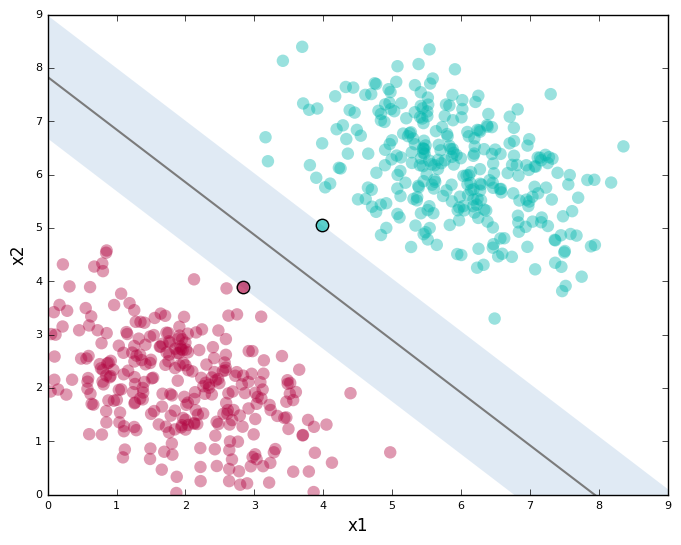Support Vector Machines give you a way to pick between many possible classifiers in a way that guarantees a higher chance of correctly labeling your test data. Pretty neat, right?

While the above plot shows a line and data in two dimensions, it must be noted that SVMs work in any number of dimensions; and in these dimensions, they find the analogue of the two-dimensional line.

For example, in three dimensions they find a plane (we will see an example of this shortly), and in higher dimensions they find a hyperplane — a generalization of the two-dimensional line and three-dimensional plane to an arbitrary number of dimensions.

Data that can be separated by a line (or in general, a hyperplane) is known as linearly separable data. The hyperplane acts as a linear classifier.

### Allowing for Errors

We looked at the easy case of perfectly linearly separable data in the last section. Real-world data is, however, typically messy. You will almost always have a few instances that a linear classifier can’t get right.

Here’s an example of such data: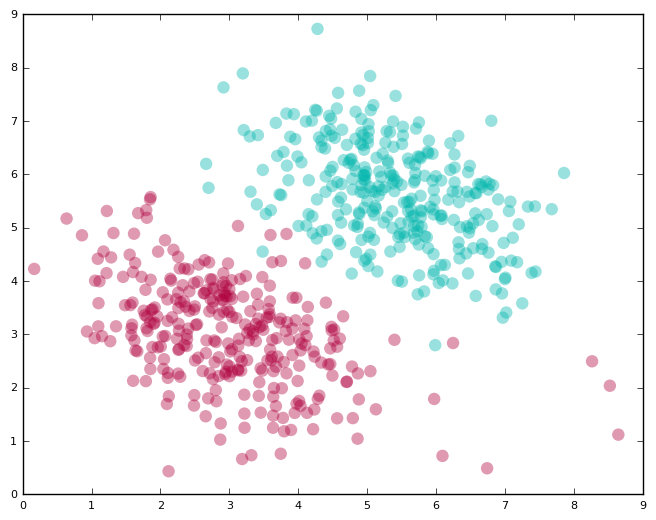Clearly, if we are using a linear classifier, we are never going to be able to perfectly separate the labels. We also don’t want to discard the linear classifier altogether because it does seem like a good fit for the problem except for a few errant points.

How do SVMs deal with this? They allow you to specify how many errors you are willing to accept.

You can provide a parameter called “C” to your SVM; this allows you to dictate the tradeoff between:

1. Having a wide margin.
2. Correctly classifying training data. A higher value of C implies you want lesser errors on the training data.

It bears repeating that this is a tradeoff. You get better classification of training data at the expense of a wide margin.

The following plots show how the classifier and the margin vary as we increase the value of C (support vectors not shown):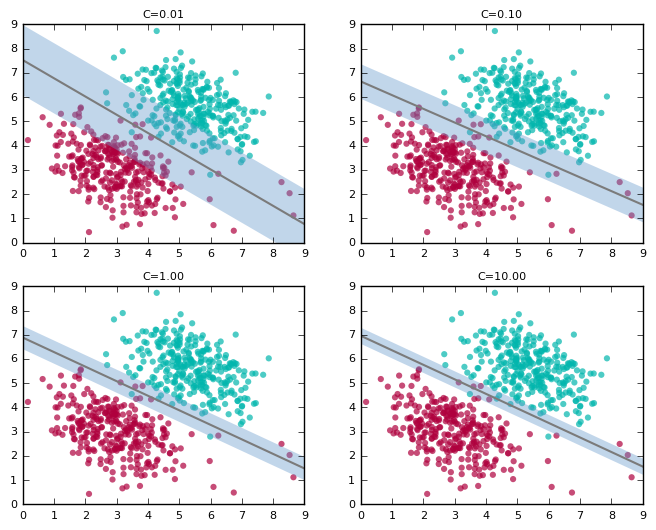Note how the line “tilts” as we increase the value of C. At high values, it tries to accommodate the labels of most of the red points present at the bottom right of the plots. This is probably not what we want for test data. The first plot with C=0.01 seems to capture the general trend better, although it suffers from a lower accuracy on the training data compared to higher values for C.

And since this is a trade-off, note how the width of the margin shrinks as we increase the value of C.

In the previous example, the margin was a “no man’s land” for points. Here, we see it’s not possible anymore to have both a good separating boundary and an associated point-free margin. Some points creep into the margin.

An important practical problem is to decide on a good value of C. Since real-world data is almost never cleanly separable, this need comes up often. We typically use a technique like cross-validation to pick a good value for C.

### Non-linearly Separable Data

We have seen how Support Vector Machines systematically handle perfectly/almost linearly separable data. How does it handle the cases where the data is absolutely not linearly separable? Afterall, a lot of real-world data falls in this category. Surely, finding a hyperplane can’t work anymore. This seems unfortunate given that SVMs excel at this task.

Here’s an example of non-linearly separable data (this is a variant of the famous XOR dataset), shown with the linear classifier SVMs find: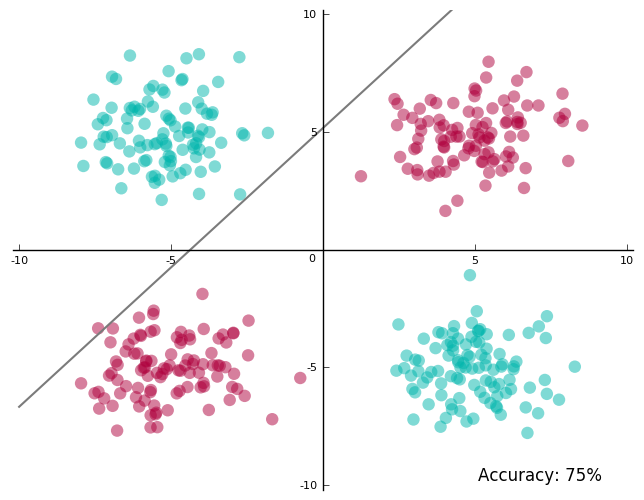You’d agree this doesn’t look great. We have only 75% accuracy on the training data — the best possible with a line. And more so, the line passes very close to some of the data. The best accuracy is not great, and to get even there, the line nearly straddles a few points.

We need to do better.

This is where one of my favorite bits about SVMs come in. Here’s what we have so far: we have a technique that is really good at finding hyperplanes. But then we also have data that is not linearly separable. So what do we do? Project the data into a space where it is linearly separable and find a hyperplane in this space!

I’ll illustrate this idea one step at a time.

We start with the dataset in the above figure, and project it into a three-dimensional space where the new coordinates are: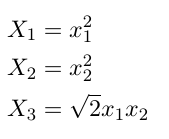This is what the projected data looks like. Do you see a place where we just might be able to slip in a plane?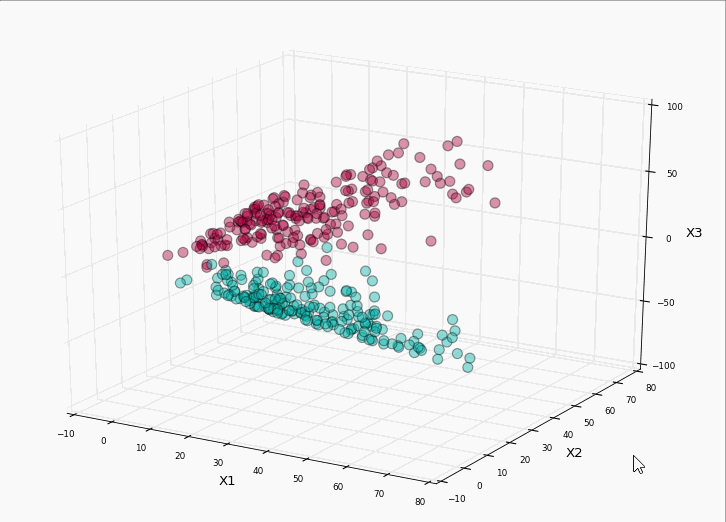Let’s run our SVM on it: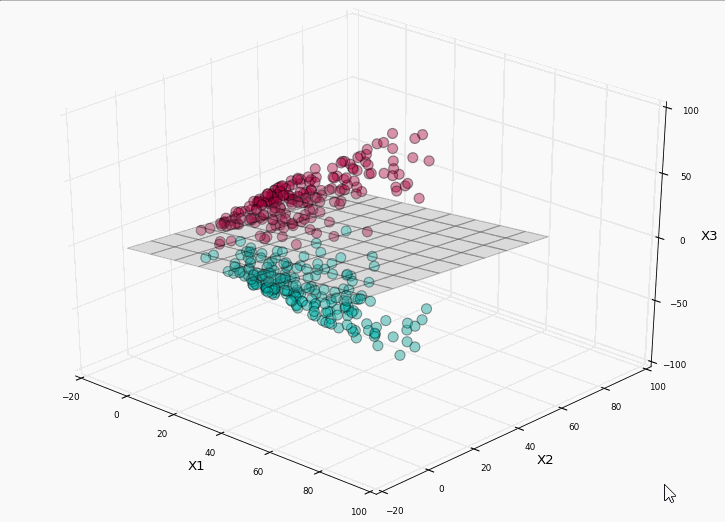Bingo! We have perfect label separation! Lets project the plane back to the original two-dimensional space and see what the separation boundary looks like: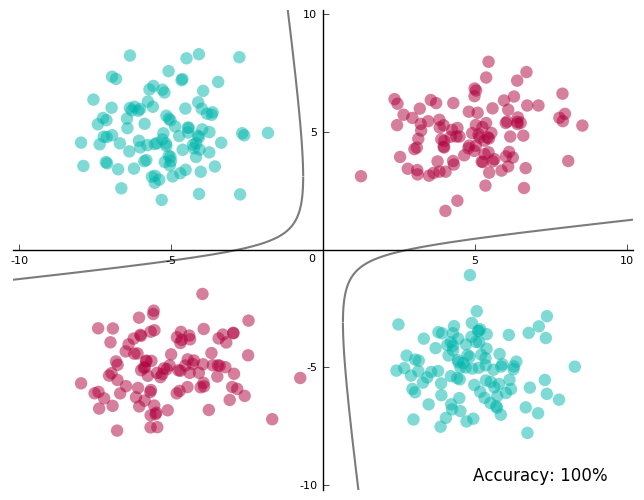100% accuracy on the training data and a separating boundary that doesn’t run too close to the data! Yay!

The shape of the separating boundary in the original space depends on the projection. In the projected space, this is always a hyperplane.

Remember the primary goal of projecting the data was to put the hyperplane-finding superpowers of SVMs to use.

When you map it back to the original space, the separating boundary is not a line anymore. This is also true for the margin and support vectors. As far as our visual intuition goes, they make sense in the projected space.

Take a look at what they look like in the projected space, and then in the original space. The 3D margin is the region (not shaded to avoid visual clutter) between the planes above and below the separating hyperplane.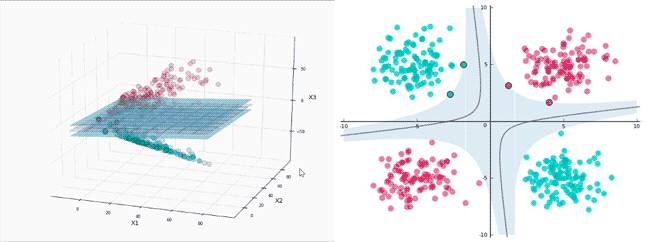There are 4 support vectors in the projected space, which seems reasonable. They sit on the two planes that identify the margin. In the original space, they are still on the margin, but there doesn’t seem to be enough of them.

Let’s step back and analyze what happened:

1. How did I know what space to project the data onto?

It seems I was being utterly specific — there is a square root of 2 in there somewhere!

In this case, I wanted to show how projections to higher dimensions work, so I picked a very specific projection. In general, this is hard to know. However, what we do know is data is more likely to be linearly separable when projected onto higher dimensions, thanks to Cover’s theorem.

In practice, we try out a few high-dimensional projections to see what works. In fact, we can project data onto infinite dimensions and that often works pretty well. This deserves going into some detail and that’s what the next section is about.

2. So I project the data first and then run the SVM?

No. To make the above example easy to grasp I made it sound like we need to project the data first. The fact is you ask the SVM to do the projection for you. This has some benefits. For one, SVMs use something called kernels to do these projections, and these are pretty fast (for reasons we shall soon see).

Also, remember I mentioned projecting to infinite dimensions in the previous point? If you project the data yourself, how do you represent or store infinite dimensions? It turns out SVMs are very clever about this, courtesy of kernels again.

It’s about time we looked at kernels.

### Kernels

Finally, the secret sauce that makes SVMs tick. This is where we need to look at a bit of math.

Let’s take stock of what we have seen so far:

1. For linearly separable data SVMs work amazingly well.
2. For data that’s almost linearly separable, SVMs can still be made to work pretty well by using the right value of C.
3. For data that’s not linearly separable, we can project data to a space where it is perfectly/almost linearly separable, which reduces the problem to 1 or 2 and we are back in business.

It looks like a big part of what makes SVMs universally applicable is projecting it to higher dimensions. And this is where kernels come in.

First, a slight digression.

A very surprising aspect of SVMs is that in all of the mathematical machinery it uses, the exact projection, or even the number of dimensions, doesn’t show up. You could write all of it in terms of the dot productsbetween various data points (represented as vectors). For p-dimensional vectors i and j where the first subscript on a dimension identifies the point and the second indicates the dimension number: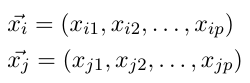The dot product is defined as: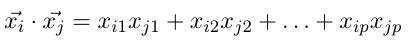If we have n points in our dataset, the SVM needs only the dot product of each pair of points to find a classifier. Just that. This is also true when we want to project data to higher dimensions. We don’t need to provide the SVM with exact projections; we need to give it the dot product between all pairs of points in the projected space.

This is relevant because this is exactly what kernels do. A kernel, short for kernel function, takes as input two points in the original space, and directly gives us the dot product in the projected space.
Let’s revisit the projection we did before, and see if we can come up with a corresponding kernel. We will also track the number of computations we need to perform for the projection and then finding the dot products — to see how using a kernel compares.

For a point i: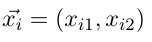Our corresponding projected point was: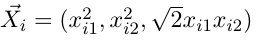To compute this projection we need to perform the following operations:

• To get the new first dimension: 1 multiplication
• Second dimension: 1 multiplication
• Third dimension: 2 multiplications

In all, 1+1+2 = 4 multiplications.

The dot product in the new dimension is: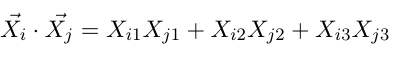To compute this dot product for two points and j, we need to compute their projections first. So that’s 4+4 = 8 multiplications, and then the dot product itself requires 3 multiplications and 2 additions.

In all, that’s:

• Multiplications: 8 (for the projections) + 3 (in the dot product) = 11 multiplications
• Additions: 2 (in the dot product)

Which is total of 11 + 2 = 13 operations.

I claim this kernel function gives me the same result: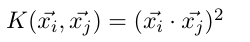We take the dot product of the vectors in the original space first, and then square the result.

Let expand it out and check whether my claim is indeed true: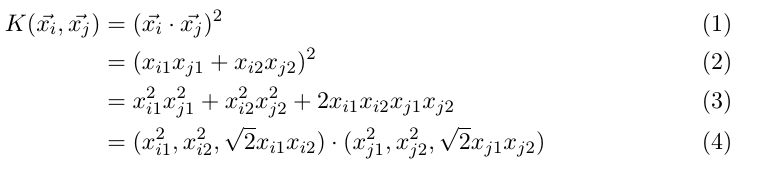It is. How many operations does this need? Look at step (2) above. To compute the dot product in two dimensions I need 2 multiplications and 1 addition. Squaring it is another multiplication.

So, in all:

• Multiplications: 2 (for the dot product in the original space) + 1 (for squaring the result) = 3 multiplications
• Additions: 1 (for the dot product in the original space)

A total of 3 + 1 = 4 operations. This is only 31% of the operations we needed before.

It looks like it is faster to use a kernel function to compute the dot products we need. It might not seem like a big deal here: we’re looking at 4 vs 13 operations, but with input points with a lot more dimensions, and with the projected space having an even higher number of dimensions, the computational savings for a large dataset add up incredibly fast. So that’s one huge advantage of using kernels.

Most SVM libraries already come pre-packaged with some popular kernels like Polynomial, Radial Basis Function (RBF), and Sigmoid. When we don’t use a projection (as in our first example in this article), we compute the dot products in the original space — this we refer to as using the linear kernel.

Many of these kernels give you additional levers to further tune it for your data. For example, the polynomial kernel: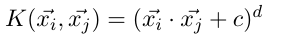allows you to pick the value of and d (the degree of the polynomial). For the 3D projection above, I had used a polynomial kernel with c=0 and d=2.

But we are not done with the awesomeness of kernels yet!

Remember I mentioned projecting to infinite dimensions a while back? If you haven’t already guessed, the way to make it work is to have the right kernel function. That way, we really don’t have to project the input data, or worry about storing infinite dimensions.

A kernel function computes what the dot product would be if you had actually projected the data.

The RBF kernel is commonly used for a specific infinite-dimensional projection. We won’t go into the math of it here, but look at the references at the end of this article.

How can we have infinite dimensions, but can still compute the dot product? If you find this question confusing, think about how we compute sums of infinite series. This is similar. There are infinite terms in the dot product, but there happens to exist a formula to calculate their sum.

1. We typically don’t define a specific projection for our data. Instead, we pick from available kernels, tweaking them in some cases, to find one best suited to the data.
2. Of course, nothing stops us from defining our own kernels, or performing the projection ourselves, but in many cases we don’t need to. Or we at least start by trying out what’s already available.
3. If there is a kernel available for the projection we want, we prefer to use the kernel, because that’s often faster.
4. RBF kernels can project points to infinite dimensions.

### SVM libraries to get started

There are quite a few SVM libraries you could start practicing with:

Many general ML libraries like scikit-learn also offer SVM modules, which are often wrappers around dedicated SVM libraries. My recommendation is to start out with the tried and tested libSVM.

libSVM is available as a commandline tool, but the download also bundles Python, Java, and Matlab wrappers. As long as you have a file with your data in a format libSVM understands (the README that’s part of the download explains this, along with other available options) you are good to go.

In fact, if you need a really quick feel of how different kernels, the value of C, etc., influence finding the separating boundary, try out the “Graphical Interface” on their home page. Mark your points for different classes, pick the SVM parameters, and hit Run!

I couldn’t resist and quickly marked a few points: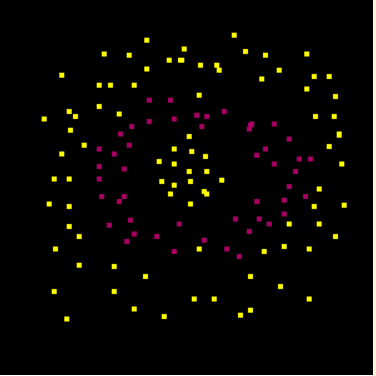Yep, I’m not making it easy for the SVM.

Then I tried out a couple of kernels: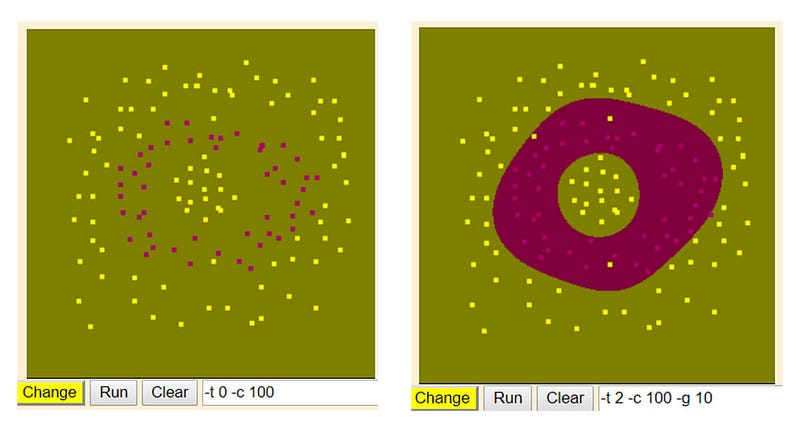The interface doesn’t show you the separating boundary, but shows you the regions that the SVM learns as belonging to a specific label. As you can see, the linear kernel completely ignores the red points. It thinks of the whole space as yellow (-ish green). But the RBF kernel neatly carves out a ring for the red label!

We have been primarily relying on visual intuitions here. While that’s a great way to gain an initial understanding, I’d strongly encourage you to dig deeper. An example of where visual intuition might prove to be insufficient is in understanding margin width and support vectors for non-linearly separable cases.

Remember that these quantities are decided by optimizing a trade-off. Unless you look at the math, some of the results may seem counter-intuitive.

Another area where getting to know the math helps is in understanding kernel functions. Consider the RBF kernel, which I’ve barely introduced in this short article. I hope the “mystique” surrounding it — its relation to an infinite-dimensional projection coupled with the fantastic results on the last dataset (the “ring”) — has convinced you to take a closer look at it.

Resources I would recommend:

1. Video Lectures: Learning from Data by Yaser Abu-Mostafa. Lectures from 14 to 16 talk about SVMs and kernels. I’d also highly recommend the whole series if you’re looking for an introduction to ML, it maintains an excellent balance between math and intuition.
2. Book: The Elements of Statistical Learning — Trevor Hastie, Robert Tibshirani, Jerome Friedman.Chapter 4 introduces the basic idea behind SVMs, while Chapter 12 deals with it comprehensively.

Happy (Machine) Learning!

Bio: Abhishek Ghose is a Senior Data Scientist at 24/7, Inc.

Original. Reposted with permission.